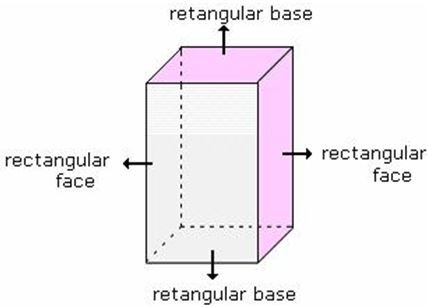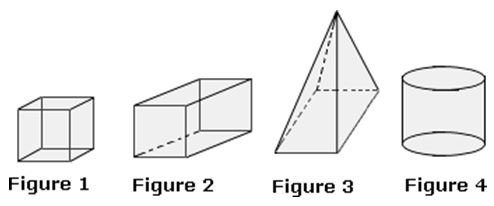## Definition Of Rectangular Prism

A prism with six rectangular faces is called a Rectangular prism

Opposite faces of a rectangular prism are equal and parallel.
A rectangular prism has 6 faces and 12 edges.
Volume of a rectangular prism = length X width X height
Surface area of a prism = 2(lw + wh + lh), where l is the length, w is the width, and h is the height of rectangular prism.

### Example of Rectangular PrismThe above solid has two rectangular bases and all its faces are rectangular.

### Solved Examples on Rectangular prism

#### Ques: Which figure is of a rectangular prism?A. Figure 1
B. Figure 2
C. Figure 3
D. Figure 4